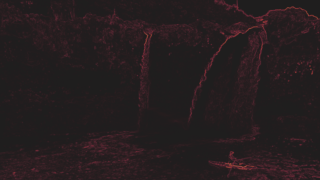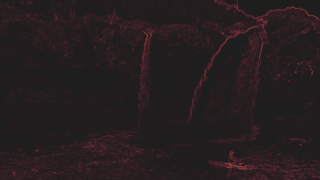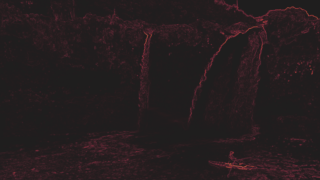## SI (Spatial Information)

#### General info

Metric type

no-reference image metric

Value range

(simple, monotone frame) 0..1 (very complex frame)

Value interpretation

bigger more complex frame

MSU VQMT implementations

MSU VQMT visualization

pixel-wise, sobel transformation

Available colorspaces

Y

Output values

metric value

Aggregated values

standard set

MSU VQMT usages

`-metr si`

ITU-T Recommendation P.910: Subjective video quality assessment methods for multimedia applications, 1999. – 37 p.

#### Algorithm description

This metric measures complexity (entropy) of an input image. This metric represents simplest SI realization that takes standard deviation of sequence of pixel values (Y-component) of Sobel transformation of input image:

$\mbox{Sobel}$ is length of vector $(\mbox{Sobel}_h, \mbox{Sobel}_v)$, where $\mbox{Sobel}_h$ and $\mbox{Sobel}_v$ are horizontal and vertical Sobel transformation. While calculating Sobel, the edge pixels will be excluded from calculation.

#### Benchmark

Choose benchmark:
Implementation System & settings Colors Resolution FPS Sec. per frame
VQMT 14.0 SI multithreaded Y HD 720p 368.29 0.004
VQMT 14.0 SI multithreaded Y FullHD 1080p 168.04 0.007
VQMT 14.0 SI multithreaded Y 4K 2160p 42.4 0.025
VQMT 14.0 SI singlethreaded Y HD 720p 81.06 0.013
VQMT 14.0 SI singlethreaded Y FullHD 1080p 36.8 0.028
VQMT 14.0 SI singlethreaded Y 4K 2160p 11.64 0.091
Measurements were done on VQMT 14.0 BETA r12792 PRO for Windows.
CPU: Intel(R) Core(TM) i7-2600 CPU @ 3.40GHz, 8 cores
GPU: NVIDIA CUDA/GeForce GTX 660 Ti
Values can vary depending on system configuration, input format and other factors
Implementation System & settings Colors Resolution FPS Sec. per frame
VQMT 13.1 SI multithreaded Y HD 720p 371.38 0.003
VQMT 13.1 SI multithreaded Y FullHD 1080p 169.24 0.007
VQMT 13.1 SI multithreaded Y 4K 2160p 42.15 0.025
VQMT 13.1 SI singlethreaded Y HD 720p 81.56 0.013
VQMT 13.1 SI singlethreaded Y FullHD 1080p 36.85 0.028
VQMT 13.1 SI singlethreaded Y 4K 2160p 11.7 0.089
Measurements were done on VQMT 13.1 r12792 PRO for Windows.
CPU: Intel(R) Core(TM) i7-2600 CPU @ 3.40GHz, 8 cores
GPU: NVIDIA CUDA/GeForce GTX 660 Ti
Values can vary depending on system configuration, input format and other factors
Implementation System & settings Colors Resolution FPS Sec. per frame
VQMT 14.0 SI multithreaded Y HD 720p 1301.78 0.002
VQMT 14.0 SI multithreaded Y FullHD 1080p 693.15 0.003
VQMT 14.0 SI multithreaded Y 4K 2160p 176.92 0.01
VQMT 14.0 SI singlethreaded Y HD 720p 83.92 0.012
VQMT 14.0 SI singlethreaded Y FullHD 1080p 39.49 0.026
VQMT 14.0 SI singlethreaded Y 4K 2160p 14.64 0.07
Measurements were done on VQMT 14.0 BETA r12792 PRO for Linux.
CPU: Intel(R) Xeon(R) Silver 4216 CPU @ 2.10GHz, 64 cores
GPU: NVIDIA CUDA/TITAN RTX
Values can vary depending on system configuration, input format and other factors

#### Example

Choose example:Original, SI-y=0.0335LQ H264, SI-y=0.0233MSU VQMT visualizationOriginal, SI-y=0.0335Blurring, SI-y=0.0102MSU VQMT visualizationOriginal, SI-y=0.0335Random points, SI-y=0.0364MSU VQMT visualizationOriginal, SI-y=0.0335Luminance shift, SI-y=0.0335MSU VQMT visualizationOriginal, SI-y=0.0335JPEG Q=2, SI-y=0.0380MSU VQMT visualizationOriginal, SI-y=0.0335JPEG Q=5, SI-y=0.0348MSU VQMT visualizationOriginal, SI-y=0.0335JPEG Q=10, SI-y=0.0338MSU VQMT visualizationOriginal, SI-y=0.0335JPEG Q=15, SI-y=0.0333MSU VQMT visualizationOriginal, SI-y=0.0335JPEG Q=20, SI-y=0.0333MSU VQMT visualizationOriginal, SI-y=0.0335JPEG Q=40, SI-y=0.0335MSU VQMT visualizationOriginal, SI-y=0.0335JPEG Q=80, SI-y=0.0336MSU VQMT visualization
Choose example:Original, SI-y=0.0626LQ H264, SI-y=0.0592MSU VQMT visualizationOriginal, SI-y=0.0626Blurring, SI-y=0.0264MSU VQMT visualizationOriginal, SI-y=0.0626Random points, SI-y=0.0649MSU VQMT visualizationOriginal, SI-y=0.0626Luminance shift, SI-y=0.0626MSU VQMT visualizationOriginal, SI-y=0.0626JPEG Q=2, SI-y=0.0718MSU VQMT visualizationOriginal, SI-y=0.0626JPEG Q=5, SI-y=0.0653MSU VQMT visualizationOriginal, SI-y=0.0626JPEG Q=10, SI-y=0.0631MSU VQMT visualizationOriginal, SI-y=0.0626JPEG Q=15, SI-y=0.0627MSU VQMT visualizationOriginal, SI-y=0.0626JPEG Q=20, SI-y=0.0626MSU VQMT visualizationOriginal, SI-y=0.0626JPEG Q=40, SI-y=0.0624MSU VQMT visualizationOriginal, SI-y=0.0626JPEG Q=80, SI-y=0.0625MSU VQMT visualization
Choose example:Original, SI-y=0.0631LQ H264, SI-y=0.0244MSU VQMT visualizationOriginal, SI-y=0.0631Blurring, SI-y=0.0203MSU VQMT visualizationOriginal, SI-y=0.0631Random points, SI-y=0.0650MSU VQMT visualizationOriginal, SI-y=0.0631Luminance shift, SI-y=0.0631MSU VQMT visualizationOriginal, SI-y=0.0631JPEG Q=2, SI-y=0.0634MSU VQMT visualizationOriginal, SI-y=0.0631JPEG Q=5, SI-y=0.0610MSU VQMT visualizationOriginal, SI-y=0.0631JPEG Q=10, SI-y=0.0602MSU VQMT visualizationOriginal, SI-y=0.0631JPEG Q=15, SI-y=0.0603MSU VQMT visualizationOriginal, SI-y=0.0631JPEG Q=20, SI-y=0.0603MSU VQMT visualizationOriginal, SI-y=0.0631JPEG Q=40, SI-y=0.0614MSU VQMT visualizationOriginal, SI-y=0.0631JPEG Q=80, SI-y=0.0624MSU VQMT visualization

#### Legacy notes

In VQMT 12 metric was optimized, consider correct range, added legacy mode.